# Long Answer Type Questions: Polynomials - Notes | Study Mathematics (Maths) Class 9 - Class 9

 1 Crore+ students have signed up on EduRev. Have you?

Question 1. Factorise: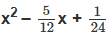Solution: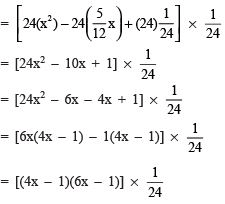Thus,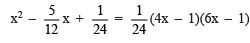Question 2. Factorise: (x6 – y6)
Solution:
x6 – y= (x3)2 – (y3)
= (x3 – y3)(x3 + y3)                                  [∵ a2 – b= (a + b)(a – b)]
= [(x – y)(x2 + xy + y2)][(x + y)(x– xy + y2)]                   [∵ a3 + b3 = (a2 + b2 – ab)(a + b) and a– b3 = (a+ ab + b2)(a – b)]
= (x – y)(x + y)(x+ xy + y2)(x2 – xy + y2)
Thus, x– y6 = (x – y)(x + y)(x2 + xy + y2)(x2 – xy + y2)

Question 3. If the polynomials 2x3 + 3x2 – a and ax3 – 5x + 2 leave the same remainder when each is divided by x – 2, find the value of ‘a’
Solution:
Let p(x) = 2x3 + 3x2 – a and f(x) = ax– 5x + 2

 when p(x) is divided by x – 2 thenremainder = p(2)since p(2) = 2(2)3 + 3(2)2 – a= 2(8) + 3(4) – a = 16 + 12 – a∴ Remainder = 28 – a when f(x) is divided by x – 2, thenremainder = f(2)since, f(2) = a(2)3 – 5(2) + 2= a(8) – 10 + 2= 8a – 8∴ Remainder = 8a – 8

28 – a = 8a – 8
⇒ 8a + a = 28 + 8
⇒ 9a = 36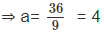Thus , a = 4

Question 4. Find the values of ‘p’ and ‘q’, so that (x – 1) and (x + 2) are the factors of x3 + 10x2 + px + q.
Solution:
Here f(x) = x+ 10x2 + px + q
Since, x + 2 = 0 [∵ x + 2 is a factor of f(x)]
⇒ x= –2 If x + 2 is a factor f(x),
then f(–2) = 0 i.e. (–2)+ 10(–2)2 + p(–2) + q = 0 [Factor theorem]
⇒ –8 + 40 + (–2p) + q = 0 ⇒ 32 – 2p + q = 0 ...(1)
⇒ 2p – q = 32 Also x – 1 = 0 ⇒ x = 1
If (x – 1) is a factor of f(x), then f(1) must be equal to 0. [Factor theorem]
i.e. (1)+ 10(1)2 + p(1) + q = 0
⇒ 1 + 10 + p + q = 0
⇒ 11 + p + q = 0
⇒ p + q = –11                                 ...(2)
Now, adding (1) and (2), we get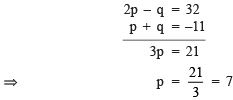Now we put p = 7 in (2), we have  7 + q = –11
⇒ q = –11 – 7 = –18
Thus, the required value of p and q are 7 and –18 respectively.

Question 5. If (x2 – 1) is a factor of the polynomial px+ qx3 + rx+ sx + t, then prove that p + r + t = q + s = 0.
Solution:
We have f(x) = px4 + qx3 + rx+ sx + t
Since, (x2 – 1) is a factor of f(x), [∵ x2 – 1 = (x + 1)(x – 1)]
then (x + 1) and (x – 1) are also factors of f(x).
∴ By factor theorem, we have   f(1) = 0 and f(–1) = 0
For f(1) = 0, p(1)4 + q(1)3 + r(1)2 + s(1) + t = 0
⇒ p + q + r + s + t = 0                       ...(1)
For f(–1) = 0, p(–1)4 + q(–1)+ r(–1)+ s(–1) + t = 0
⇒ p – q + r – s + t = 0                     ...(2)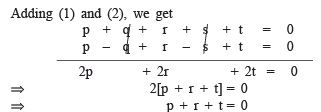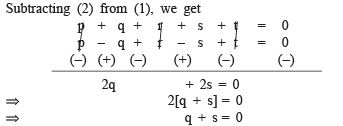From (4) and (3), we get p + r + t = q + s = 0

Question 6. If x + y = 12 and xy = 27, find the value of x3 + y3.
Solution:
Since, (x + y)3 = x3 + y3 + 3xy (x + y)
∴ Substituting x + y = 12 and xy = 27,
we have: (12)3 =x+ y3 + 3 (27) (12)
⇒ x3 + y3 = 81(12) – 123
= [92 – 122] × 12
= [(9 + 12) (9 – 12)] × 12
= 21 × 3 × 12 = 756

Question 7. If a + b + c = 5 and ab + bc + ca = 10, Then prove that a+ b3 + c3 – 3abc = –25.
Solution:
Since, a3 + b3 + c– 3abc
= (a + b + c) (a+ b2 + c– ab – bc – ca)
∴ a3 + b3 + c3 – 3 abc = (a + b + c) [(a2 + b2 + c2 + 2ab+ 2bc+ 2cb) − 3ab − 3bc − 3ca]
= (a + b + c) [(a + b + c)2 − 3 (ab + bc + ca)]
= 5 [ 52 − 3(10)]
= 5[25 – 30]
= 5[–5] = –25

Question 8. If a, b, c are all non-zero and a + b + c = 0, prove that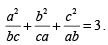Solution:
Since, a + b + c = 0
∴ a3 + b3 + c3 = 3abc                         ..... (1)
Now, in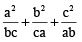= 3, we have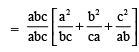[Multiplying and dividing by ‘abc’]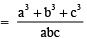..... (2)

From (1) and (2), we have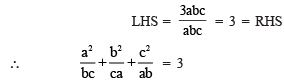The document Long Answer Type Questions: Polynomials - Notes | Study Mathematics (Maths) Class 9 - Class 9 is a part of the Class 9 Course Mathematics (Maths) Class 9.
All you need of Class 9 at this link: Class 9

## Mathematics (Maths) Class 9

88 videos|397 docs|109 tests
 Use Code STAYHOME200 and get INR 200 additional OFF

## Mathematics (Maths) Class 9

88 videos|397 docs|109 tests

Track your progress, build streaks, highlight & save important lessons and more!

,

,

,

,

,

,

,

,

,

,

,

,

,

,

,

,

,

,

,

,

,

;